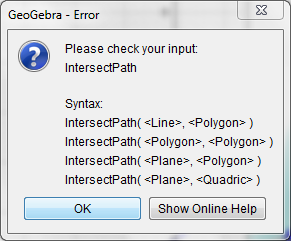# Why can't I graph the intersection of a Sphere and Cylinder?

Evan O'Leary shared this problem 4 years ago
Not a Problem

I'm trying to use Intersect() to graph the intersection of a sphere centered a the origin, and a cylinder with half the radius whose circle slices are centered at a point halfway between the sphere's center and its circumference.

But it keeps giving me an undefined error. Is there a problem with the system?1

not implemented1

You can solve algebraicly in CAS, but it gives you also a false solution. You will have to fiddle with a list of parametric equations.1

Here is the equation of this intersection. Solving it by hand gives a better result. Solving for x gives

x=r-(z^2)/r you can then solve for y with the sphere equation (2 values). z is the parameter.